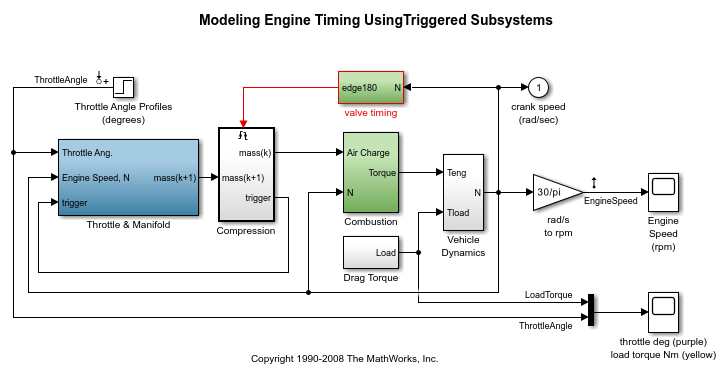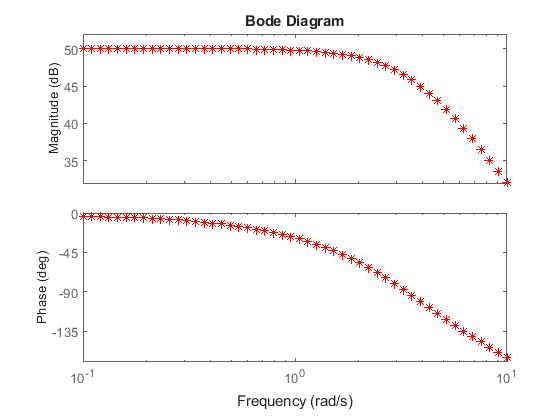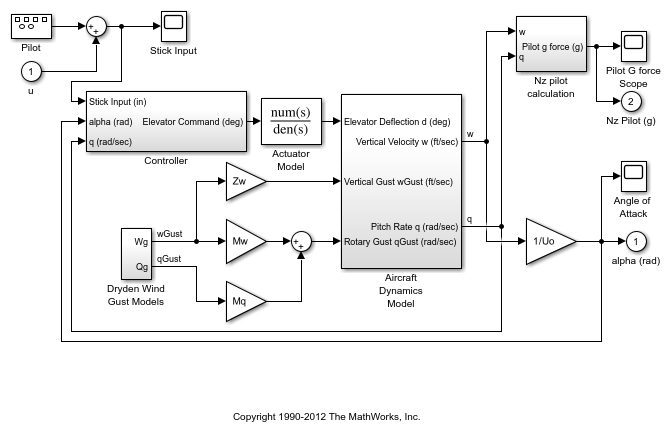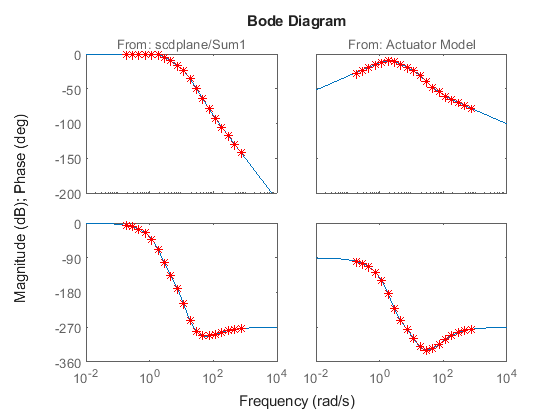# Speed Up Frequency Response Estimation Using Parallel Computing

This example illustrates how to speed up frequency response estimation of Simulink® models using parallel computing. In some scenarios, the `frestimate` function estimates the frequency response of a Simulink model by performing multiple Simulink simulations. You can distribute these simulations to a pool of MATLAB® workers by using Parallel Computing Toolbox™ software.

This example requires Parallel Computing Toolbox software. You can optionally run simulations on a computer cluster using MATLAB Parallel Server™ software. This example uses the local worker functionality available in Parallel Computing Toolbox software.

### Speed up Simulink Simulations Performed by `frestimate`

When you compute a frequency response using the `frestimate` function, the majority of computation time is spent in Simulink simulations. To reduce the total simulation time, you can:

1. Use rapid accelerator mode. Use this method when `frestimate` performs only one Simulink simulation. For an example, see Validate Linearization in Frequency Domain at Command Line.

2. Distribute simulations across workers in a MATLAB pool. Use this method when `frestimate` performs multiple Simulink simulations. `frestimate` performs more than one Simulink simulation when you specify the following:

• A sinestream input signal with the `SimulationOrder` parameter set to `'OneAtATime'`. In this case, each frequency in the sinestream signal is simulated separately.

• Linear analysis points with more than one input point or a nonscalar input point. In this case, each linearization input point or each channel in a nonscalar linearization input point yields a separate Simulink simulation.

Using the `frestimate` function with parallel computing also supports normal, accelerator, and rapid accelerator modes.

### Configure a MATLAB Pool

To use parallel computing to speed up frequency response estimation, configure and start a pool of MATLAB workers before you run the `frestimate` function.

To check if a MATLAB pool is open, use the `gcp` function. If no pool is open, open one using the `parpool` function.

```if isempty(gcp) parpool local end ```

### Distribute Simulink Simulations for Each Frequency in Sinestream Input

When you use a sinestream input signal with the `frestimate` function and you set the `SimulationOrder` parameter to `'OneAtATime'`, each frequency in the sinestream signal simulates in a separate Simulink simulation. If you enable the parallel computing option, the simulations corresponding to individual frequencies are distributed among workers in the MATLAB pool.

Open the model, and obtain the linear analysis points stored in the model.

```mdl = 'scdengine'; open_system(mdl) io = getlinio(mdl); ```Create a sinestream input signal with a `'OneAtATime'` simulation order.

```in = frest.Sinestream('Frequency',logspace(-1,1,50),'Amplitude',1e-3,... 'SimulationOrder','OneAtATime'); ```

In this model, there is a single linearization input point and a single linearization output point. There are 50 frequencies in the sinestream signal. The `frestimate` command performs 50 separate Simulink simulations because the `SimulationOrder` parameter is set to `'OneAtATime'`.

To distribute these simulations among workers, enable parallel computing for `frestimate`. Create an `frestimateOptions` object and set the `UseParallel` option to `'on'`. Use this object as an input argument for `frestimate`.

```opt = frestimateOptions('UseParallel','on'); sysest = frestimate(mdl,io,in,opt); bode(sysest,'r*') ```In general, parallel computing significantly speeds up frequency response estimation using `frestimate`. The actual processing times and amount of improvement will depend on your computer setup and your Parallel Computing Toolbox configuration. For example, the amount of improvement can be affected by various factors including the overhead from client-to-worker data transfer and resource competition between worker processes and OS processes.

Close the model.

```bdclose(mdl) ```

### Distribute Simulink Simulations for Input Channels

When the number of linearization input points or the number of channels in a linearization input point is greater than one, the `frestimate` command distributes individual Simulink simulations corresponding to these input channels among workers in the MATLAB pool.

Open the model, and obtain the linear analysis points stored in the model.

```mdl = 'scdplane'; open_system(mdl) io(1) = linio('scdplane/Sum1',1,'input'); io(2) = linio('scdplane/Actuator Model',1,'input'); io(3) = linio('scdplane/Gain5',1,'output'); ```With the `linio` function, you specify two linearization input points, which are both located on scalar Simulink signals. If you run the `frestimate` command to estimate the frequency response for this model, two Simulink simulations occur, one for each input.

Linearize the model, and create an input signal using the linearization result.

```sys = linearize(mdl,io); in = frest.Sinestream(sys); ```

Before estimating the frequency response, find all source blocks in the signal paths of the linearization outputs that generate time-varying signals using the `findSources` function. Such time-varying signals can interfere with the signal at the linearization output points and produce inaccurate estimation results. To disable the time-varying source blocks, create an `frestimateOptions` option set and specify the `BlocksToHoldConstant` option.

```srcblks = frest.findSources('scdplane',io); opt = frestimateOptions('BlocksToHoldConstant',srcblks); ```

Enable parallel computing using the `UseParallel` estimation option, which distributes simulations among workers.

```opt.UseParallel = 'on'; ```

Run `frestimate` using parallel computing.

```sysest = frestimate(mdl,io,in,opt); ```

Plot the estimation result against the analytical linearization

```bodeopts = bodeoptions; bodeopts.PhaseMatching = 'on'; bodeplot(sys,sysest,'r*',bodeopts); ```Close the model, the open figure, and the parallel pool.

```bdclose(mdl) close(gcf) delete(gcp) ```